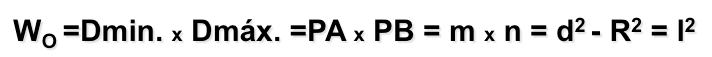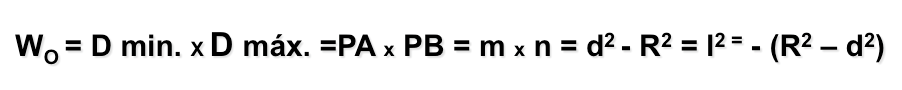# Metric geometry : Concepto de “Potencia de un punto respecto de una circunferencia”

We will use the concepts of arc capable of a segment at our shows, what is suggested by his review.
This concept is based on product of two segments and, but as we shall see adelante, to determine some important loci such as radical axis of two circles.

## Definition of power

The first definition of power is based on determining the minimum and maximum distance to a circle and get your product metric.
Power W to a point P on a circumference c product is the most for the least distance from the point P the circumference c.

The figure shows that the power point P respect to the circumference is product segmentsm” and “n“, minimum and maximum distance from the point to the circumference. These segments are located on the diameter of the circle containing point P.

## Performance metrics Relations

We can relate metrically the basic concept of power on a circumference, using the Pythagorean theorem, tangency with the segment is obtained from the point to the circumference.

The power of a point P on a circumference is equal to the square difference between the distance from point P center C the circumference and the radius R de la SMA; also the square of the segment PT the Tangente say P is outside.If we consider the segment “m” equals the distance “d” Point “P” center “C” the circumference “c“, minus the radius “R” de la SMA (d-R), and segment “n” is the sum of “d” and “R” (d R) we must:As the sum of two times the difference variable is the difference of the squares, We see that the power “W” is equal to the difference of the squares of the distance “d” and the radius “R” the circumference. This expression reminds the leg of a right triangle, whose square is equal to the difference of squares of the hypotenuse and the other leg (side the).

If the point P is internal to the circumference of the segment not exist tangency, but we can also establish the relationship with the sides of a Pythagorean triangle.The power of a point P on a circumference is equal to the difference of squares of the distance from point P center C the circumference and the radius R thereof and also to the square of half chord segment PT perpendicular a PC you P is internal.Power point (Wikipedia)